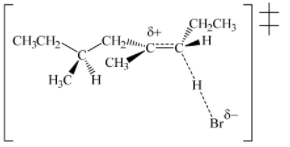# Problem: For the transition state structure below, choose an organic reaction in which it is involved and give a chemical equation for your selected organic reaction that includes the following: (i) structural formula(s) for the organic reactant(s); be sure to show stereochemistry appropriately when necessary (ii) the experimental conditions (Give structural formulas for organic compounds. Give a chemical formula or inorganic reactants or catalysts . If heat and/or light is needed, be sure to indicate it appropriately.) (iii) structural formula(s) for the major organic product(s); be sure to show stereochemistry appropriately when necessary. As we do for most organic reaction equations, the chemical equations that you give do not need to be balanced.

97% (89 ratings)
###### Problem Details

For the transition state structure below, choose an organic reaction in which it is involved and give a chemical equation for your selected organic reaction that includes the following:

(i) structural formula(s) for the organic reactant(s); be sure to show stereochemistry appropriately when necessary

(ii) the experimental conditions (Give structural formulas for organic compounds. Give a chemical formula or inorganic reactants or catalysts . If heat and/or light is needed, be sure to indicate it appropriately.)

(iii) structural formula(s) for the major organic product(s); be sure to show stereochemistry appropriately when necessary. As we do for most organic reaction equations, the chemical equations that you give do not need to be balanced.Frequently Asked Questions

What scientific concept do you need to know in order to solve this problem?

Our tutors have indicated that to solve this problem you will need to apply the E1 Reaction concept. You can view video lessons to learn E1 Reaction. Or if you need more E1 Reaction practice, you can also practice E1 Reaction practice problems.

What is the difficulty of this problem?

Our tutors rated the difficulty ofFor the transition state structure below, choose an organic ...as medium difficulty.

How long does this problem take to solve?

Our expert Organic tutor, Jonathan took 5 minutes and 38 seconds to solve this problem. You can follow their steps in the video explanation above.

What professor is this problem relevant for?

Based on our data, we think this problem is relevant for Professor Tsvetkov's class at FSU.